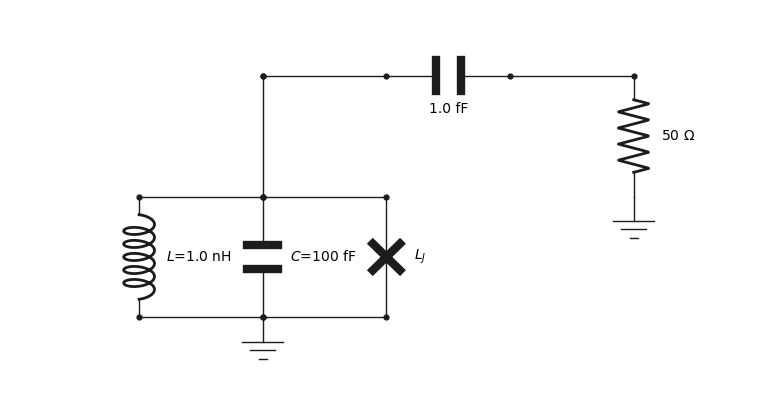# Graphical user interface¶

class qucat.GUI(filename, edit=True, plot=True, print_network=True)[source]

Opens a graphical user interface to constructs a circuit.

Parameters
• filename (string) – Path to a file which will store all the information about the graphically constructed circuit.

• edit (Boolean) – If True (default), the graphical user interface will be opened. One can set this argument to False to import the circuit without opening the graphical user interface

• plot (Boolean) – If True (default), the circuit will be plotted using matplotlib.

• print_network (Boolean) – If True (default), a text description of the constructed network will be printed.

Returns

A Qcircuit object, see qucat.Qcircuit

Return type

qucat.Qcircuit

Notes

All the necessary information about the circuit generated by the graphical user interface application is stored in a human-readable format at the specified path.

Each line of this text file is in the format:

type ; x_minus , y_minus ; x_plus , y_plus ; value ; label

and represents a circuit component, wire or ground element.

type can take the values L, C, R, J, W or G for inductor, capacitor, resistor, junction, wire or ground respectively.

value will be a float representing the value of the component or will be empty

label will be a string corresponding to the label of the component or will be empty

x/y_minus (x/y_plus) represents the horizontal/vertical location of the minus (plus) node of the component. Negative value are allowed and components have a length of 1 unit.

For example, the circuit below, is described by the following text file

L;3,-10;3,-11;1.000000e-09;L
C;4,-10;4,-11;1.000000e-13;C
J;5,-10;5,-11;;L_J
C;5,-12;6,-12;1.000000e-15;
R;7,-11;7,-12;5.000000e+01;
G;7,-10;7,-11;;